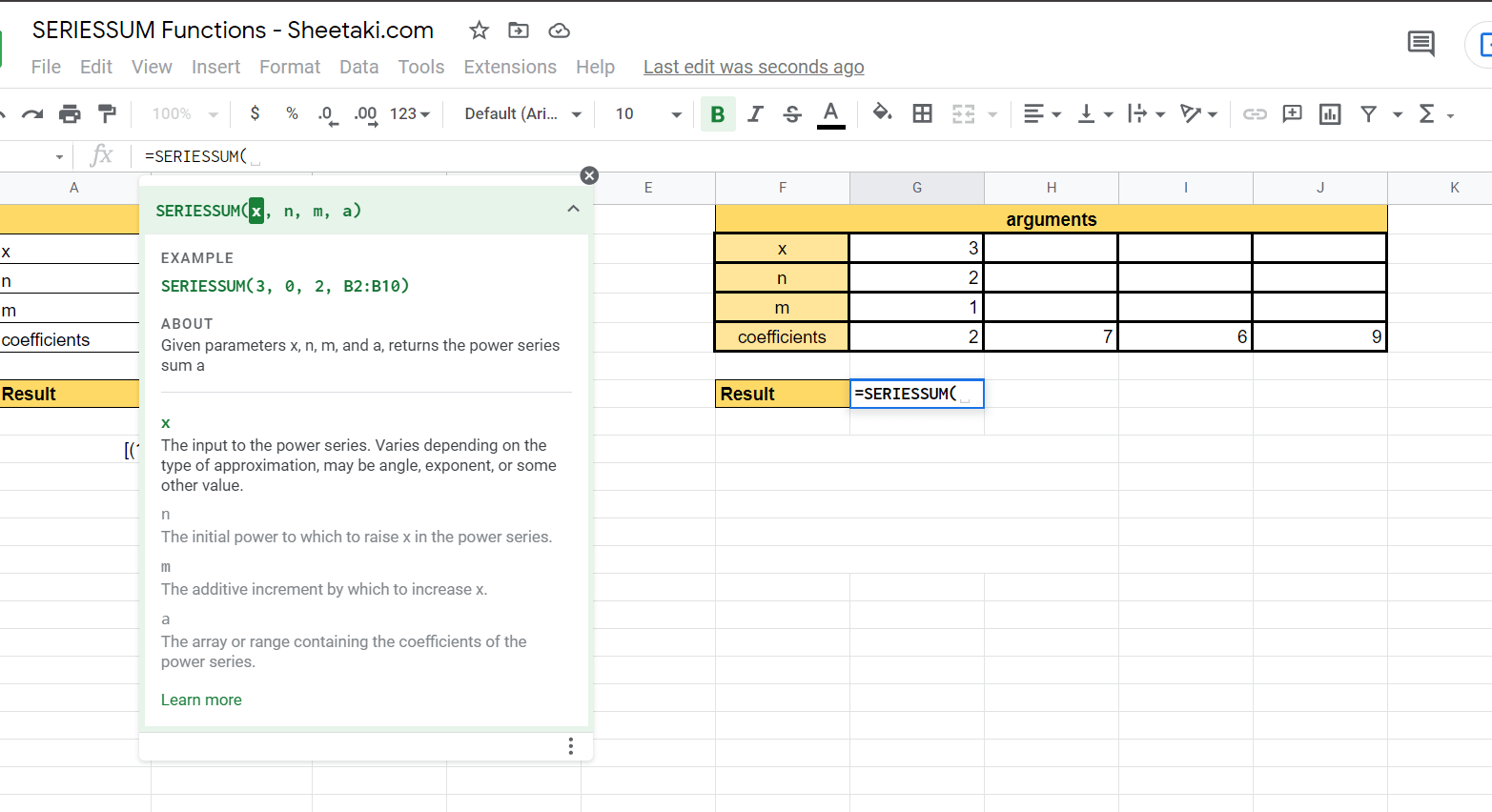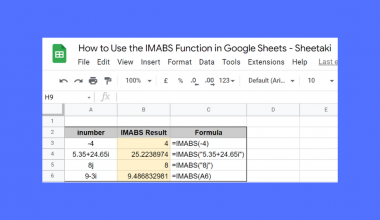# How To Use SERIESSUM Function in Google Sheets

The SERIESSUM function in Google Sheets is useful when you need to compute the sum of a power series.

Wee can use a power series to approximate various mathematical constants and functions. However, this function is mostly used in the context of user-defined models.

The rules for using the `SERIESSUM` function in Google Sheets are as follows:

• The function requires four arguments that determine the values to input in the power series.
• The function then outputs a number which is the sum of every element in the indicated power series.

Let’s take a look at a quick demonstration of this function in use.

Let’s say we would like to approximate the value of Pi/4 radians using a power series. We know that each term has the following coefficients (1, -0.5, 0.04167, -0.0001389) and a constant of pi/4 (0.78539…). The first term is raised to the power of 0, and each term increments that power by 2. How do we determine the value of this power series?

With the `SERIESSUM` function, it becomes quite easy to solve this without having to type the entire formula yourself, which might become a tedious process for the user.

This use case is just one of many ways to use the `SERIESSUM` function in Google Sheets.

Let’s learn how to write the `SERIESSUM` function ourselves in Google Sheets and later test out the function with actual date values.

## The Anatomy of the SERIESSUM Function

The syntax of the `SERIESSUM` function is as follows:

`=SERIESSUM(x, n, m, a)`

Let’s dissect this thing and understand what each of these terms means:

• = the equal sign is how we start any function in Google Sheets.
• SERIESSUM() is our `SERIESSUM` function. It computes the sum of a power series.
• x refers to the input to the power series.
• n refers to initial power to which x must be raised.
• m refers to the additive increment.
• a refers to the values of the coefficients of the power series. It may be in the form of an array or a cell range.

## A Real Example of Using SERIESSUM Function

Let’s have a look at an example of the `SERIESSUM` function being used in a Google Sheets spreadsheet.

In the table below, we have our four arguments laid out on a table. Using the `SERIESSUM` function, we were able to come up with 12 as a result.To get the value in cell B7, we just need to type in the following formula:

`=SERIESSUM(B2,B3,B4,B5:D5)`

Let’s confirm whether this is the right answer.

In the worksheet below, we go step-by-step into how to compute the sum of the power series. Since there are three coefficients, we’ll be having three terms. Each term has a corresponding coefficient (shown in parentheses) and a constant raised to some power. The power is indicated by the argument n and grows for every succeeding term by m. Since n=1 and m=0, the power remains 1 for every term.Computing for the final answer gives us 2+4+6=12 as the final simplified equation.

In the example below, we have a different set of arguments. Manually computing for the series shows that `SERIESSUM` has produced the right result.Let’s start writing `SERIESSUM` function in Google Sheets. Head over to the next section to begin our step-by-step guide on how to use the function.

## How to Use SERIESSUM Function in Google Sheets

1. First, we must set up the arguments for our `SERIESSUM` function. As seen below, we must have values for n, m, x, and a.2. To start using the `SERIESSUM` function, select the cell we will first put our function’s output. In this example, we’ll place our final result in cell G7.3. Next, we just simply type the equal sign ‘=‘ to begin the function, followed by ‘SERIESSUM(‘.
4. You may encounter a tooltip box with information about the `SERIESSUM` function. This box can be minimized by clicking on the arrow in the top-right-hand side.5. Next, we need to type in our arguments. In this case, our arguments are already placed in the proper order. We should write the last argument as a range.
Afterward, simply hit Enter on your keyboard to let the function evaluate.1. Why does my formula return a #VALUE! error?
The `SERIESSUM` function only accepts numeric values. Non-numeric values will result in a #VALUE! error.
2. How many terms can be in my power series?
The number of terms in your power series is determined by the size of your coefficient array. If your array has a length of 20, then your power series will likewise have 20 terms.

That’s everything you need to know about the `SERIESSUM` function in Google Sheets. This step-by-step guide shows how easy it is to set up your own power series for your own custom models.

You may now use the `SERIESSUM` function in Google Sheets together with the various other Google Sheets formulas available to build powerful spreadsheet solutions.

If you found this article useful, you can subscribe to our newsletter to be the first to know about the latest guides and tutorials from us.

[powerkit_subscription_form title=”Get emails from us about Google Sheets.” text=”Our goal this year is to create lots of rich, bite-sized tutorials for Google Sheets users like you. If you liked this one, you’d love what we are working on! Readers receive ✨ early access ✨ to new content.” list_id=”default” bg_image_id=”2256″

##### You May Also Like## Remove Duplicates from Comma-Delimited Strings in Google Sheets

In this article, you will learn how you can use a simple formula to remove duplicates from comma-delimited…## How to Use MINVERSE Function in Google Sheets

The MINVERSE function in Google Sheets is useful when you need to compute the multiplicative inverse of a…## How to Append Text to Each Cell in A Column in Google Sheets

Google Sheets has several functions in its arsenal to manipulate text which is useful when you need to…## How to Use the IMABS Function in Google Sheets

The IMABS function in Google Sheets returns the absolute value (the modulus) of a complex number. A complex…